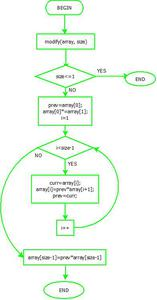# Replace every array element by multiplication of previous and next

Given an array of integers, update every element with the multiplication of previous and next elements with the following exceptions.

a) The First element is replaced by the multiplication of the first and second.
b) The last element is replaced by multiplication of the last and second last.

Example:

```Input  : arr[] = {2, 3, 4, 5, 6}
Output : arr[] = {6, 8, 15, 24, 30}```
```// We get the above output using following
// arr[] = {2*3, 2*4, 3*5, 4*6, 5*6} ```
`Source: Top 25 Interview Questions`

Simple Solution is to create an auxiliary array ay, copy the contents of the given array to the auxiliary array. Finally, traverse the auxiliary array and update the given array using copied value. The Time complexity of this solution is O(n), but it requires O(n) extra space.
An efficient solution can solve the problem in O(n) time and O(1) space. The idea is to keep track of previous elements in the loop.

Flowchart:Flowchart- modify

Algorithm:

• Create a method named “modify” that takes an integer array “arr” and its length “n” as input parameters.
• now check if the length is less than or equal to 1, if yes then return.
• Create a variable named “prev” and initialize it with the first element of the array.
• Then change the value of the first element of the array with the product of the first and second elements.
•  Start a for loop and traverse the array from the second element to the second last element
• In a loop, Create a variable named “curr” and initialize it with the value of the current element of the array.
•  Now, update the current element of the array with the product of the previous and next elements.
•  Set the value of “prev” with the value of “curr”.
•  Now out of the loop, update the value of the last element of the array with the product of the second last, and last elements.

Below is the implementation of this idea.

## C++

 `// C++ program to update every array element with` `// multiplication of previous and next numbers in array` `#include` `using` `namespace` `std;`   `void` `modify(``int` `arr[], ``int` `n)` `{` `    ``// Nothing to do when array size is 1` `    ``if` `(n <= 1)` `      ``return``;`   `    ``// store current value of arr and update it` `    ``int` `prev = arr;` `    ``arr = arr * arr;`   `    ``// Update rest of the array elements` `    ``for` `(``int` `i=1; i

## Java

 `// Java program to update every array element with` `// multiplication of previous and next numbers in array` `import` `java.io.*;` `import` `java.util.*;` `import` `java.lang.Math;`   `class` `Multiply` `{` `   ``static` `void` `modify(``int` `arr[], ``int` `n)` `    ``{` `        ``// Nothing to do when array size is 1` `        ``if` `(n <= ``1``)` `            ``return``;`   `        ``// store current value of arr and update it` `        ``int` `prev = arr[``0``];` `        ``arr[``0``] = arr[``0``] * arr[``1``];`   `        ``// Update rest of the array elements` `        ``for` `(``int` `i=``1``; i

## Python3

 `# Python program to update every array element with` `# multiplication of previous and next numbers in array`   `def` `modify(arr, n):`   ` ``# Nothing to do when array size is 1` `    ``if` `n <``=` `1``:` `        ``return`   `    ``# store current value of arr and update it` `    ``prev ``=` `arr[``0``]` `    ``arr[``0``] ``=` `arr[``0``] ``*` `arr[``1``]`   `    ``# Update rest of the array elements` `    ``for` `i ``in` `range``(``1``, n``-``1``):` `    `  `        ``# Store current value of next interaction` `        ``curr ``=` `arr[i];`   `        ``# Update current value using previous value` `        ``arr[i] ``=` `prev ``*` `arr[i``+``1``]`   `           ``# Update previous value` `        ``prev ``=` `curr` `    `    `    ``# Update last array element` `    ``arr[n``-``1``] ``=` `prev ``*` `arr[n``-``1``]`     `# Driver program` `arr ``=` `[``2``, ``3``, ``4``, ``5``, ``6``]` `n ``=` `len``(arr)` `modify(arr, n)` `for` `i ``in` `range` `(``0``, n):` `    ``print``(arr[i],end``=``" "``)`   `# This code is contributed by` `# Smitha Dinesh Semwal`

## C#

 `// C# program to update every array ` `// element with multiplication of` `// previous and next numbers in array` `using` `System;`   `class` `GFG ` `{` `    ``static` `void` `modify(``int` `[]arr, ``int` `n)` `    ``{` `        ``// Nothing to do when array size is 1` `        ``if` `(n <= 1)` `            ``return``;`   `        ``// store current value of arr and update it` `        ``int` `prev = arr;` `        ``arr = arr * arr;`   `        ``// Update rest of the array elements` `        ``for` `(``int` `i=1; i

## PHP

 ``

## Javascript

 ``

Output

`6 8 15 24 30 `

Time complexity: O(n) where n is size of given array
Auxiliary Space: O(1) because it is using constant space for variables